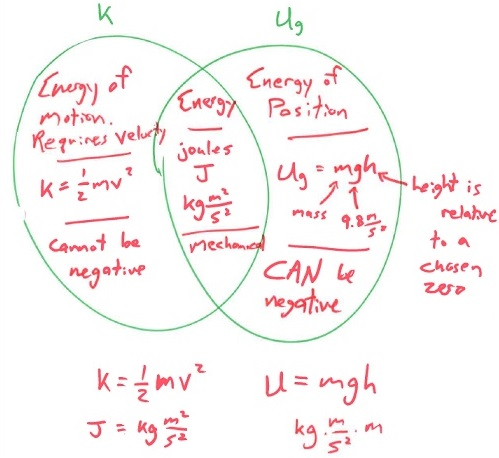# Venn Diagram of Kinetic and Potential Energies

1 teachers like this lesson
Print Lesson

## Objective

Students compare and contrast kinetic energy and potential energy by creating a Venn Diagram of the two types of energy.

#### Big Idea

Kinetic energy is the energy of motion, potential energy is the energy of position.

## Context

This is a shorted period due to a weather delay, so the total time for this lesson is 30 minutes instead of the usual 50 minutes. I had planned on students using computer simulators to determine how the shape of a ramp affects the energy transformations from potential to kinetic, but this is pushed off to the next lesson. Instead, we review the homework and then students do a compare/contrast activity where they make a Venn diagram for kinetic and potential energy.

NGSS HS-PS3-2 is applied to this activity as students use models to illustrate that energy at the macroscopic scale can be accounted for as a combination of energy associated with the motions of objects and energy associated with the relative positions of objects. The review of the homework assignment is a quantitative application of conservation of energy which aligns with NGSS Science Practice 5: Using mathematics and computational thinking and Science Practice 7: Engaging in argument from evidence. This also applies CCSS Math Practice 2Reason abstractly and quantitatively and Math Practice 3: Construct viable arguments.

## Mouse Roller Coaster Review

5 minutes

As the class begins the solutions to the Mouse roller coaster solution from the previous lesson are projected on the board. The students are expected to check and correct their own work with a different colored pen/pencil. The solutions show the equations solved for the required variables (kinetic energy equation solved for velocity and potential energy equation solved for height). I expect my students to be able to manipulate the equations to solve for those unknowns and show them how to do this.

Then, I call on random students to answer these questions such as: Where is the velocity the greatest? Where is the potential energy the largest? What happens to the kinetic/potential energy if there is friction present? This is to activate their thinking for the activities that are coming up the next lesson.

## Review Energy Homework Problems

10 minutes

I instruct students to take out their homework Energy Practice Problems assigned last class for review. I post the Energy Practice Problem - Solutions (download this document to see both pages) with a document camera and instruct students to check their work and fix mistakes. I go around and check that students completed the assignment. If they complete both sides and show work, they get full credit. While I check their work, I scan their sheets to see if there are common problems or mistakes students make so that I can review those concepts.

There are different ways to use homework, sometimes I assess student understanding of the topic by giving a quiz, sometimes I collect the homework and grade it for correctness. The purpose of this assignment is to have students practice the application of the kinetic energy and potential energy formulas, which they first learned yesterday; I had checked student understanding then.

After I enter a homework grade for all of the students, I return to the front of the room and address some of the common errors I that students have on their homework sheet. I also ask what questions students have on the homework so that they can clear up any misunderstandings.

## Kinetic versus Potential - Compare and Contrast

15 minutes

With not enough time to do my planned activity, I decide to have students create a Venn diagram in their notebooks. They can work collaboratively on this activity, but every student is expected to make a diagram in their notebook. When students identify similarities and differences between two related concepts, they are forced to organize various aspects of the concepts and figure out how they are related. The visual tool of a Venn diagram is a useful tool to accomplish this task and allows students to direct their own classification. There are a variety of items that can be placed in the diagrams and students have to be able to justify what they put into it. This is an application of CCSS Math Practice 3: Construct viable arguments and critique the reasoning of others.

Students have 5 minutes to fill in their Venn diagrams with as many things as they can think of. I tell them that they should have at least nine similarities/difference on their chart, three per part. While students do this, I project and start the online timer so that they know exactly how much time they have to complete the task. I find that when I give students an exact amount of time to complete a task, they tend to be more focused.When the time is up, I use my document camera and project missing word diagram on the whiteboard. I then call on random students to supply what they put in their Venn diagrams. I make is clear that if students do have have one of the items I write down on their own diagram, they are to include it.

This activity is a great exercise to fortify the concepts of kinetic and potential energy. This is important to do as students use these concepts extensively in the coming lessons. Because of this, I inform students that they should memorize everything on these diagrams in preparation for the next lessons where we revisit the energy skate park.